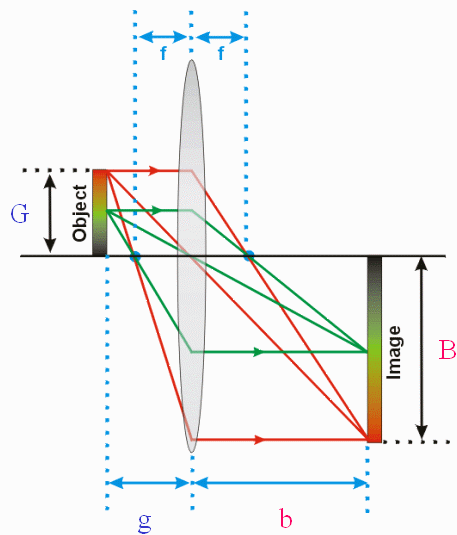Summary of the Presentation:

Diagrams and equations

A summary of thin lens (TL) diagram.

Click on the diagram to open in its own window that includes a reference table that defines the variables.TL equations

1) 1/f = 1/b + 1/g

2) m= B/G = b/g (m = magnification) If m < 1, then the image is actually reduced.

Determining the controlling dimension

Width to height ratios are equal for both the scene and image: (WS / HS) = (WC / HC), then G = either WS or HS

Width is the controlling dimension: (WS / HS) >(WC / HC), then G = WS

Height is the controlling dimension: (WS / HS) < (WC / HC), then G = HS

B will always equal the corresponding variable for the image: WC or HC

Formative evaluation - lesson presentationFormative evaluation #2: This is a quick survey on your impression of the lesson presentation, including the review.

Note: you only have to enter your real name at the top of the survey. There is no ID number, and the e-mail address is optional.

Now you are ready to solve some practice problems.